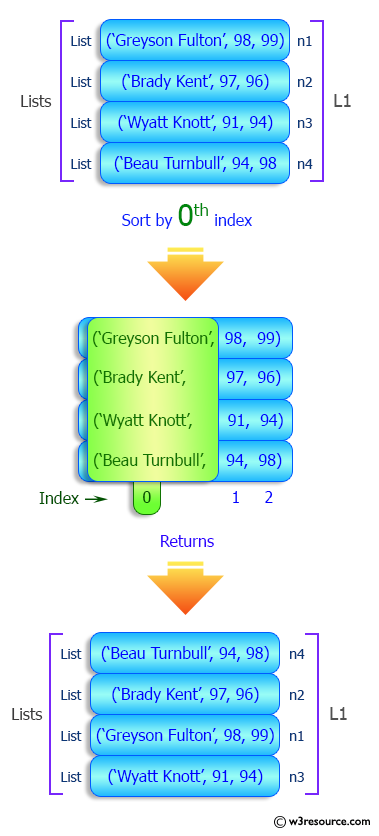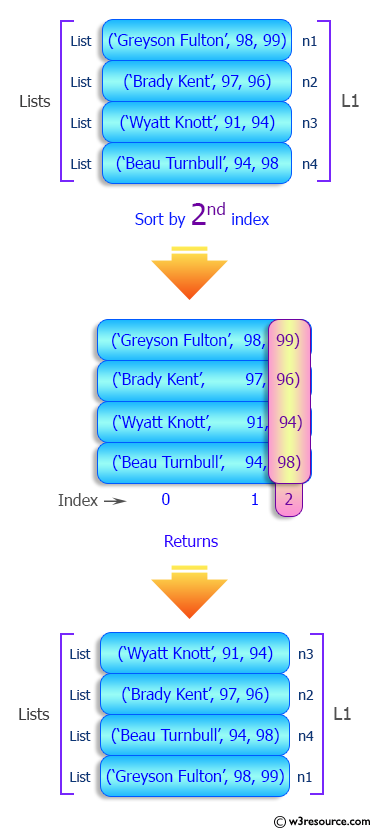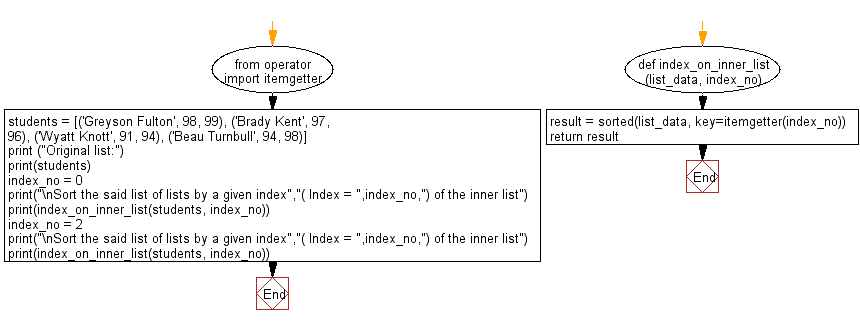﻿ Python: Sort a list of lists by a given index of the inner list - w3resource

# Python: Sort a list of lists by a given index of the inner list

## Python List: Exercise - 116 with Solution

Write a Python program to sort a list of lists by a given index of the inner list.

Sample Solution:

Python Code:

``````from operator import itemgetter
def index_on_inner_list(list_data, index_no):
result = sorted(list_data, key=itemgetter(index_no))
return result
students = [('Greyson Fulton', 98, 99), ('Brady Kent', 97, 96), ('Wyatt Knott', 91, 94), ('Beau Turnbull', 94, 98)]
print ("Original list:")
print(students)
index_no = 0
print("\nSort the said list of lists by a given index","( Index = ",index_no,") of the inner list")
print(index_on_inner_list(students, index_no))
index_no = 2
print("\nSort the said list of lists by a given index","( Index = ",index_no,") of the inner list")
print(index_on_inner_list(students, index_no))
```
```

Sample Output:

```Original list:
[('Greyson Fulton', 98, 99), ('Brady Kent', 97, 96), ('Wyatt Knott', 91, 94), ('Beau Turnbull', 94, 98)]

Sort the said list of lists by a given index ( Index =  0 ) of the inner list
[('Beau Turnbull', 94, 98), ('Brady Kent', 97, 96), ('Greyson Fulton', 98, 99), ('Wyatt Knott', 91, 94)]

Sort the said list of lists by a given index ( Index =  2 ) of the inner list
[('Wyatt Knott', 91, 94), ('Brady Kent', 97, 96), ('Beau Turnbull', 94, 98), ('Greyson Fulton', 98, 99)]
```

Pictorial Presentation:Flowchart:## Visualize Python code execution:

The following tool visualize what the computer is doing step-by-step as it executes the said program:

Python Code Editor:

Have another way to solve this solution? Contribute your code (and comments) through Disqus.

What is the difficulty level of this exercise?

Test your Programming skills with w3resource's quiz.

﻿

## Python: Tips of the Day

Returns True if the provided function returns True for every element in the list, False otherwise:

Example:

```def tips_every(lst, fn=lambda x: x):
return all(map(fn, lst))

print(tips_every([2, 4, 3], lambda x: x > 1))
print(tips_every([1, 2, 3]))
```

Output:

```True
True
```